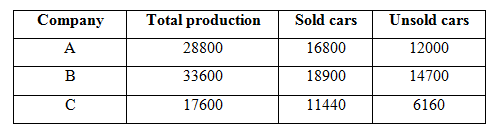# SBI Clerk Prelims 2021 Quantitative Aptitude Questions (Day-45)

Dear Aspirants, Our IBPS Guide team is providing new series of Quantitative Aptitude Questions for SBI Clerk Prelims 2020 so the aspirants can practice it on a daily basis. These questions are framed by our skilled experts after understanding your needs thoroughly. Aspirants can practice these new series questions daily to familiarize with the exact exam pattern and make your preparation effective.

Start Quiz

Caselet

Directions (01-05): Study the following information carefully and answer the given questions?

There are 3 different companies A, B and C, producing cars. It has a total production of 80000. The percentage of total production of A and B is 36 % and 42 % respectively. The ratio of sold to that of unsold cars of company A and C is 7: 5 and 13: 7 respectively. The unsold cars of company B is 2100 less than the sold cars of company A.

1) Find the difference between the total sold cars of company B to the total production of company C?

A.1300

B.1500

C.1700

D.1400

E.None of these

2) Find the ratio between the total number of cars sold by company A to that of company B?

A.18: 7

B.25: 9

C.8: 9

D.42: 23

E.None of these

3) Find the difference between the average number of sold cars of company A and B together and the average number of unsold cars of the same companies?

A.3400

B.4200

C.4500

D.3700

E.None of these

4) Total production of company B is approximately what percentage of total unsold cars of company A and C together?

A.170 %

B.185 %

C.155 %

D.200 %

E.130 %

5) Find the total sold cars of company A, B and C together?

A.49360

B.45520

C.43780

D.47140

E.None of these

Simplification

Directions (06-10): What value should come in the place of (?) in the following questions?

6) 4? × (225)1/2 = 248 ÷ 8 + 929

A.2

B.4

C.3

D.5

E.None of these

7) 450 ÷ 15 + 208 ÷ 8 – 16 = ?2 – 41

A.8

B.9

C.7

D.6

E.None of these

8) 256(1/8) * 642 = 16a

A.a = 13/4

B.a = 12/5

C.a = 11/3

D.a = 13/3

E.a = 11/4

9) √4489 + √169 +? = 19 * 24

A.328

B.359

C.364

D.376

E.386

10) 767 ÷ 13 + 357 ÷ √289 =? * √16

A.10

B.15

C.20

D.25

E.30

Directions (1-5) :

Total production of company A, B and C = 80000

Total production of company A = 80000*(36/100) = 28800

Total production of company B = 80000*(42/100) = 33600

Total production of company C = 80000*(22/100) = 17600

The sold cars of company A = 28800*(7/12) = 16800

The unsold cars of company A = 28800 – 16800 = 12000

The sold cars of company C = 17600*(13/20) = 11440

The unsold cars of company C = 17600 – 11440 = 6160

The unsold cars of company B = 16800 – 2100 = 14700

The sold cars of company B = 33600 – 14700 = 18900The total sold cars of company B = 18900

The total production of company C = 17600

Required difference = 18900 – 17600 = 1300

The total number of cars sold by company A = 16800

The total number of cars sold by company B = 18900

Required ratio = 16800: 18900 = 8: 9

The average sold cars of company A and B together

= > (16800 + 18900)/2 = 17850

The average unsold cars of company A and B together

= > (12000 + 14700)/2 = 13350

Required difference = 17850 – 13350 = 4500

Total production of company B = 33600

Total unsold cars of company A and C together

= > 12000 + 6160 = 18160

Required % = (33600/18160) * 100 = 185 %

The total sold cars of company A, B and C together

= > 16800 + 18900 + 11440

= > 47140

4x × (225)1/2 = 248 ÷ 8 + 929

4x * 15 = (248/8) + 929

4x = (31 + 929)/15

4x = 64

4x = 43

x = 3

450 ÷ 15 + 208 ÷ 8 – 16 = x2 – 41

(450/15) + (208/8) – 16 + 41 = x2

30 + 26 – 16 + 41 = x2

x2 = 81

x = 9

256(1/8) * 642 = 16a

44/8 * 43 * 2  = 42a

1/2 + 6 = 2a

13/4 = a

√4489 + √169 +? = 19 * 24

67 + 13 +? = 456

? = 376

767 ÷ 13 + 357 ÷ √289 =? * √16

59 + 21 =? * 4

? = 20

 Check Here to View SBI Clerk Prelims 2021 Quantitative Aptitude Questions Day – 44 Day – 43 Day – 42